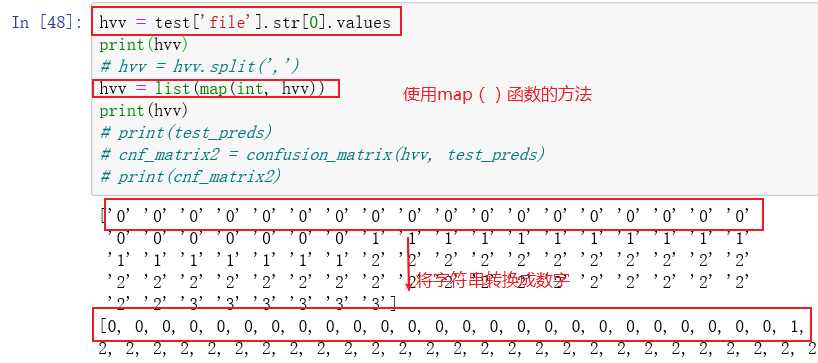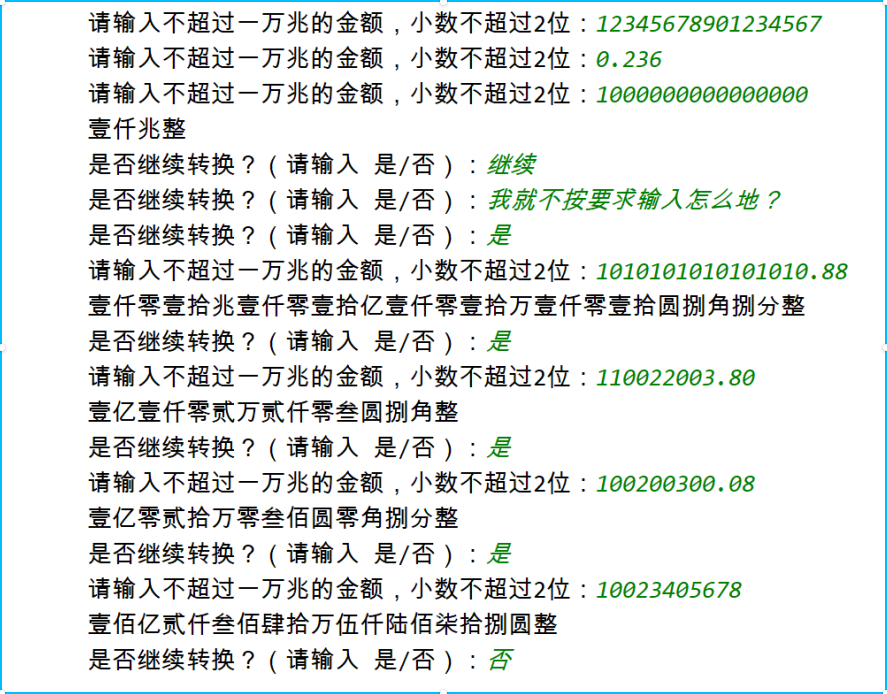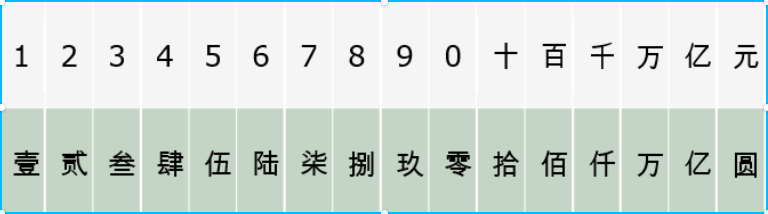• 本文实例讲述了Python列表元素转为数字的方法。分享给大家供大家参考，具体如下： 有一个数字字符的列表： 1 ... numbers = ['1', '5', ... 想要把每个元素转换为数字： 1 numbers = [...
原文链接：https://www.jb51.net/article/86561.htm

本文实例讲述了Python中列表元素转为数字的方法。分享给大家供大家参考，具体如下：

有一个数字字符的列表：

1

numbers = ['1', '5', '10', '8']

想要把每个元素转换为数字：

1

numbers = [1, 5, 10, 8]

用一个循环来解决：

1

2

3

4

new_numbers = [];

for n in numbers:

  new_numbers.append(int(n));

numbers = new_numbers;

有没有更简单的语句可以做到呢？

1.

1

numbers = [ int(x) for x in numbers ]

2. Python2.x,可以使用map函数

1

numbers = map(int, numbers)

如果是3.x,map返回的是map对象，当然也可以转换为List:

1

numbers = list(map(int, numbers))

3.还有一种比较复杂点：

1

for i, v in enumerate(numbers): numbers[i] = int(v)


展开全文• 本文实例讲述了Python列表元素转为数字的方法 分享给大家供大家参考，具体如下： 1.首先可以用循环来解决（直接...想要把每个元素转换为数字： numbers = [2, 5, 130, 8] 用一个循环来解决： new_numbers = [];...
本文实例讲述了Python中列表元素转为数字的方法
分享给大家供大家参考，具体如下：
1.首先可以用循环来解决（直接粗暴）
举个栗子：
<1>有一个数字字符的列表：
numbers = ['2', '5', '130', '8']

<2>想要把每个元素转换为数字：
numbers = [2, 5, 130, 8]

用一个循环来解决：
new_numbers = [];
for n in numbers:
new_numbers.append(int(n));
numbers = new_numbers;

2.有没有更简单的语句可以做到呢？
1.使用列表生成式
.numbers = [ int(x) for x in numbers ]

2. Python2.x,可以使用map函数
numbers = map(int, numbers)

3.如果是3.x,map返回的是map对象，当然也可以转换为List:
numbers = list(map(int, numbers))

4..还有一种比较复杂点：
for i, v in enumerate(numbers): numbers[i] = int(v)

额外（遇到的问题补充<踩坑>）：
如何将pandas Series或index转换为Numpy数组？
1.要获取NumPy数组，您应该使用以下values属性：
In : df = pd.DataFrame({'A': [1, 2, 3], 'B': [4, 5, 6]}, index=['a', 'b', 'c']); df
A  B
a  1  4
b  2  5
c  3  6

In : df.index.values

Out: array(['a', 'b', 'c'], dtype=object)

2.这可以访问数据的存储方式，因此无需进行转换。
注意：此属性也可用于许多其他pandas的对象。
In : df['A'].values

Out: Out: array([1, 2, 3])

3.要将索引作为列表，请调用tolist：
In : df.index.tolist()

Out: ['a', 'b']

同样，对于列。
编码踩坑的纪实展开全文字符串 numpy series
• 想要把每个元素转换为数字： numbers = [2, 4, 1, 3] 1. Python2.x，可以使用map函数： numbers = map(int, numbers) 2. Python3.x，map返回的是map对象，当然也可以转换为List: numbers = list(map(int, numbers...
• ## python将列表中的字符串转为数字

万次阅读 多人点赞 2019-06-05 21:14:05
python 将列表中的字符串转为数字 ... 本文实例讲述了Python中列表元素转为数字的方法。分享给大家供大家参考，具体如下： 有一个数字字符的列表： ... 想要把每个元素转换为数字： 1 ...
python 将列表中的字符串转为数字

转自：https://www.jb51.net/article/86561.htm

本文实例讲述了Python中列表元素转为数字的方法。分享给大家供大家参考，具体如下：

有一个数字字符的列表：

1

numbers = ['1', '5', '10', '8']

想要把每个元素转换为数字：

1

numbers = [1, 5, 10, 8]

用一个循环来解决：

1

2

3

4

new_numbers = [];

for n in numbers:

  new_numbers.append(int(n));

numbers = new_numbers;

有没有更简单的语句可以做到呢？

1.

1

numbers = [ int(x) for x in numbers ]

2. Python2.x,可以使用map函数

1

numbers = map(int, numbers)

如果是3.x,map返回的是map对象，当然也可以转换为List:

1

numbers = list(map(int, numbers))

3.还有一种比较复杂点：

for i, v in enumerate(numbers): numbers[i] = int(v)

python字符数字之间的转换：转自https://www.cnblogs.com/wuxiangli/p/6046800.html

int(x [,base ])         将x转换为一个整数

long(x [,base ])        将x转换为一个长整数

float(x )               将x转换到一个浮点数

complex(real [,imag ])  创建一个复数

str(x )                 将对象 x 转换为字符串

repr(x )                将对象 x 转换为表达式字符串

eval(str )              用来计算在字符串中的有效Python表达式,并返回一个对象

tuple(s )               将序列 s 转换为一个元组

list(s )                将序列 s 转换为一个列表

chr(x )                 将一个整数转换为一个字符

unichr(x )              将一个整数转换为Unicode字符

ord(x )                 将一个字符转换为它的整数值

hex(x )                 将一个整数转换为一个十六进制字符串

oct(x )                 将一个整数转换为一个八进制字符串

chr(65)='A'

ord('A')=65

int('2')=2;

str(2)='2'


展开全文• 题目 python练习的一个小实验, 题目如下图: ...3. 整数部分可以分割以 4 位数字为一组的列表来进行转换处理，这样便于进行 （兆，亿，万，圆）。的单位处理 4. 首先分割的 4 位数字一组的数据进行转化处理，便...
题目

python练习的一个小实验,  题目如下图:附阿拉伯数字对应的中文大写：思路

1. 通过定义一个字典进行阿拉伯数字与大写中文的对比储存
2. 将用户的输入首先分割为整数与小数部分分别进行处理
3. 整数部分可以分割为以 4 位数字为一组的列表来进行转换处理，这样便于进行
（兆，亿，万，圆）。的单位处理
4. 首先将分割的 4 位数字一组的数据进行转化处理，便于对 （拾，佰，仟）的单
位进行处理（此部分应编写到一个函数之中，接受的参数为 4 位数的字符串，
返回转化好的字符串）
5. 整数部分可以看做多个 4 位数字一组的数据处理，通过遍历列表进行转化处理
（例如你已将 123456789 分割好为[‘1’,’2345’,’6789’],循环遍历这个列表， 用第
四点提到的函数进行转化，遍历转化时把【兆，亿，万，圆】的单位标识添加）
6. 通过这种方式进行转化你需要定义两个列表分别储存（兆，亿，万，圆）和
（拾，佰，仟）这些单位标识
7. 小数部分的处理通过分支判断情况进行转化即可。

代码

思路清晰了, 代码直接码上即可, 注意处理特殊条件和边界:

#!/usr/bin/env python
# -*- coding: utf-8 -*-
# @File  : num_to_chinese.py
# @Author: meta
# @Contact : metashen@qq.com
# @Date  : 20-3-28下午9:48
# @Desc  : 阿拉伯数字代表的金额， 转换为大写数字

limit_len1 = 16  # 不超过一万兆
limit_len2 = 2  # 小数位不超过两位

dictionary = {
'0': '零',
'1': '壹',
'2': '贰',
'3': '叁',
'4': '肆',
'5': '伍',
'6': '陆',
'7': '柒',
'8': '捌',
'9': '玖'
}

level1 = {
1: '圆',
2: '万',
3: '亿',
4: '兆'
}

level2 = {
2: '拾',
3: '佰',
4: '仟'
}

level3 = {
1: '角',
2: '分'
}

def query():
while 1:
ans = str(input('请输入不超过一万兆的金额, 小数位不超过两位: '))
if len(ans.split('.')) <= limit_len1 \
and len(ans.split('.')) <= limit_len2:
return ans

def process_integer(num_integer):
pre = []
length = len(num_integer)
if length <= 4:
pre.append(num_integer)
else:
extra = length % 4
if extra != 0:
pre.append(num_integer[0: extra])
length = length - extra
start, end = extra, 4 + extra
else:
start, end = 0, 4
while length:
pre.append(num_integer[start: end])
start += 4
end += 4
length -= 4
return pre

def per_conver(num):
length = len(num)
flag = True

# Special
# deal with '0000'
if num == '0000':
return ''
# deal with '1000'
if num[1:] == '000':
return dictionary[num] + level2

# Normal
res, p = '', length
for i in range(0, length):
# deal with prefix is '0'
if (res == '' or res == dictionary[num[i]]) and num[i] == '0':
# only one '0'
if flag:
res += dictionary[num[i]]
flag = False
p -= 1
continue
# deal with '1001'
if i + 1 < length and num[i + 1] == '0' and num[i] == '0':
p -= 1
continue
# deal with '1010' '1110'
if i == length - 1 and num[i] == '0':
continue
res += dictionary[num[i]]
if p > 1 and num[i] != '0':
res += level2[p]
p -= 1
return res

def convert_integer(ans_int):
res = ''
p = len(ans_int)
for i in ans_int:
per_res = per_conver(i)
res += per_res
if p > 0 and per_res != '':
res += level1[p]
p -= 1
return res

def convert_float(num_float):
res = ''
for i in range(0, len(num_float)):
res += dictionary[num_float[i]]
if (i + 1) <= 2:
res += level3[i + 1]
return res

def convert():
number = query()
res = ''
if '.' in number:
num_split = number.split('.')
num_integer = num_split
num_float = num_split
ans_int = process_integer(num_integer)
print(ans_int)
# Convert integer
res += convert_integer(ans_int)
# Convert float
res += convert_float(num_float)
else:
ans_int = process_integer(number)
res += convert_integer(ans_int)
res += '整'
print(res)

if __name__ == '__main__':
convert()

为了校验正确性, 附上数字金额转中文大写金额的在线工具:  https://szjrzzwdxje.51240.com/
`
展开全文• 1.code numb1 = input('输入数字：').split() print(numb1) numb2 = list(map(int, numb1)) print(numb2) 2.result 输入数字：1 2 3 54 ['1', '2', '3', '54'] [1, 2, 3, 54]算法
• python整数转换字符串Given a string with digits and we have to convert the string to its ... 给定一个带有数字的字符串，我们必须该字符串转换为Python中与之等效的整数列表。 Example: 例： Input: s...字符串 c++ java
• 今天刷题，碰到了一个需要将数字倒序输出的...在Python里，输入数字转化字符串后，用str()函数，倒序可以用reversed()函数，辅以list()可直接得到数字对应的list。接着可以用该代码： output = int("".join(m...
• 在做机器学习项目时，经常要将列表中不同字符换为不同数字，比如： ['Jogging' 'Jogging' 'Jogging' 'Jogging' 'Jogging' 'Jogging' 'Upstairs' 'Upstairs' 'Downstairs' 'Downstairs' 'Downstairs'] 转换为 [1 ...numpy
• 分享给大家供大家参考，具体如下：有一个数字字符的列表：numbers = ['1', '2', '3', '4']想要把每个元素转换为数字：numbers = [1, 2, 3, 4]1. Python2.x，可以使用map函数：numbers = map(int, numbers)2. Python3...
• 将数字分解成列表 ...那么问题转换成如何读取一个数的每一个数字，我们可以对数字进行取余，然后再将数字进行降位数，如下代码： a = num % 10 #余数即最后一个数字 b = num // 10 #在python中需要要到地
• 法1： number = ['1', '2', '3', '4'] number = [int(x) for x in number] print(number);...number = list(map(int, number)) # 使用内置map返回一个map对象，再用list转换为列表 print(number) 更...
• import xlrd as xd data =xd.open_workbook ('1.xlsx') #打开excel...for r in range(sheet.nrows): #表中数据按行逐步添加到列表中，最后转换为list结构 data1 = [] for c in range(sheet.ncols): data1.append.
• 一. 数字与字符串 int(x [,base ]) x转换为一个整数 long(x...
• 函数str():让python将非字符串值转换为字符串： age = 23 message = "Happy " + str(age) + "rd Birthday!" print(message) 列表 列表是由一系列按特定顺序排列的元素组成，可将任意东西加入列表中 在Python中，用...
• 这是一个实用程序，Python使用十六进制数字字符串并转换为单词列表。 十六进制数字必须成对-这是一个可被二整除的字符串。 实时版本，用于生成密码 这是一个JS页面，可在四个或更多单词的本地JS页面中生成...
• 将字符串转换成列表 str1 =‘high voltage capacitors,high energy capacitor’ str1.split(’,’) ==>...将列表转换成字符串，用join(list())、join(tuple())函数 当列表的值为数字时,不能使用join...
• s1=list(map（int，s2）) 可以s2里面的符合规定的字符串数字转换为数字 s2是列表 s1也是链表 list（str）可以直接转换str为一个一个的字母并放在list中 str（list）不改变原有list，无法直接转换 列表转字符串需要...
• 这个对象可以是纯数字的字符串，也可以是浮点数，如 int('10') 结果为10，int(10.6) 结果为10hex()：用于十进制数字转换成十六进制，如 hex(10) 结果为'0xa'str()：用于将数字转换为字符串，如 str(10) 结果为 '10......

# python将列表转换为数字python 订阅